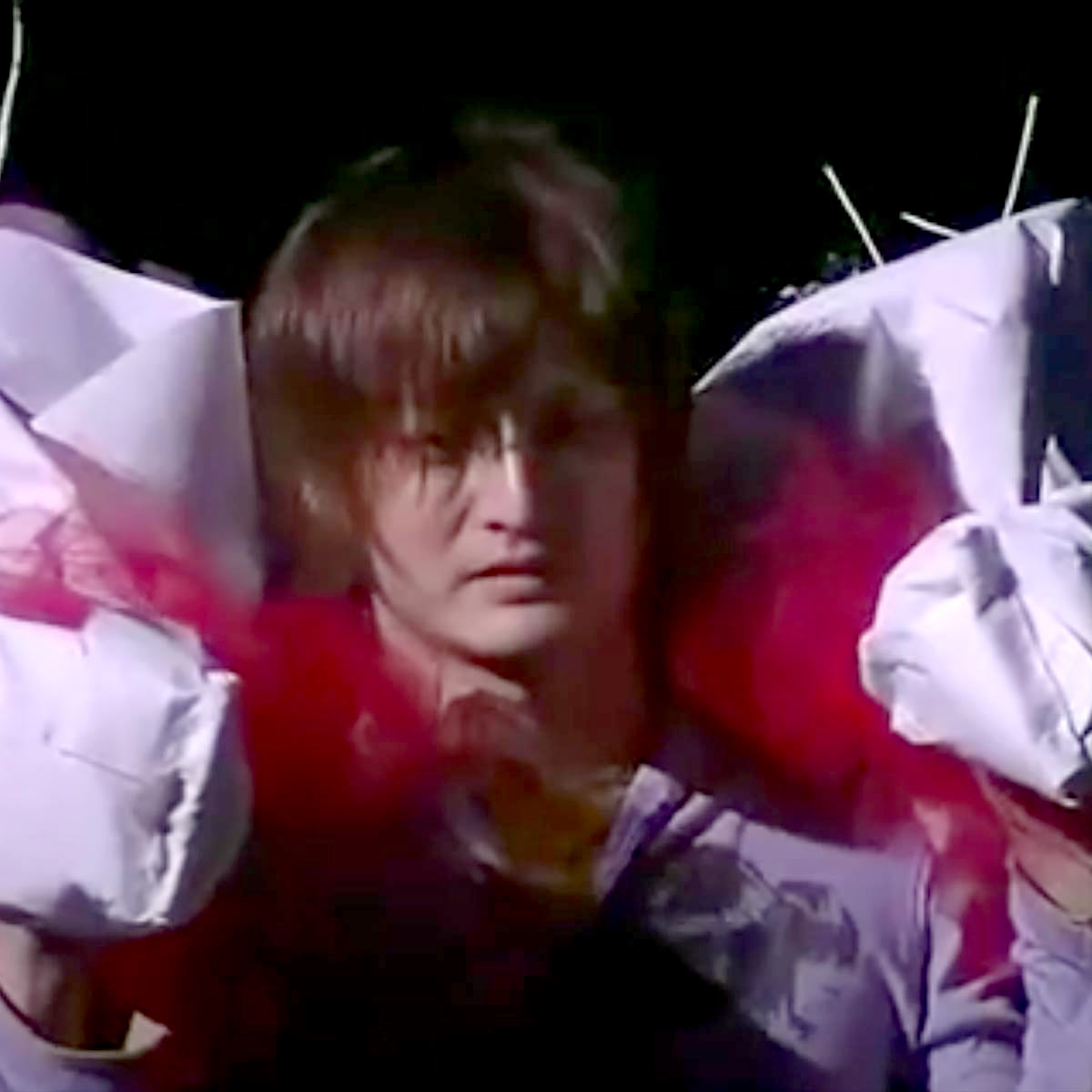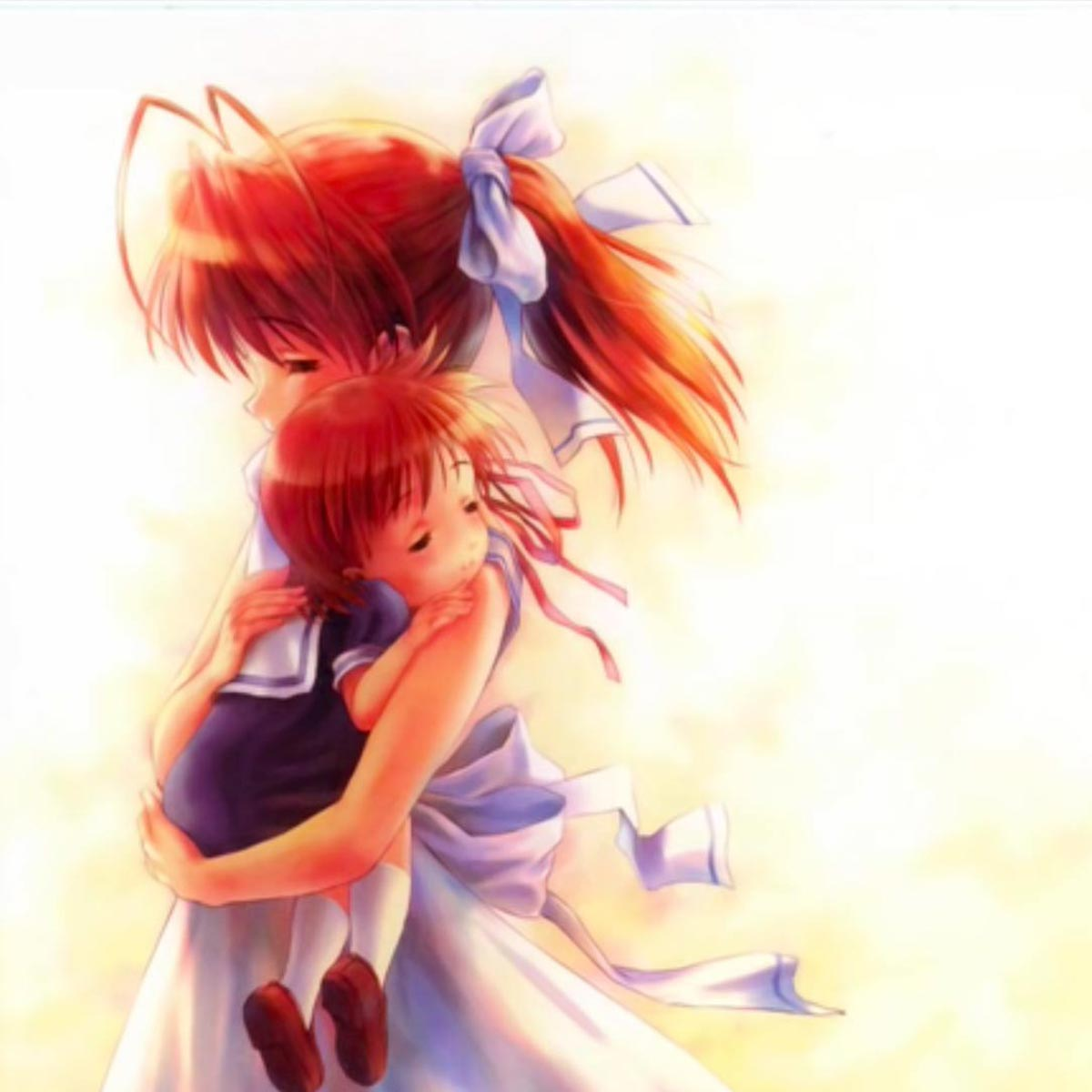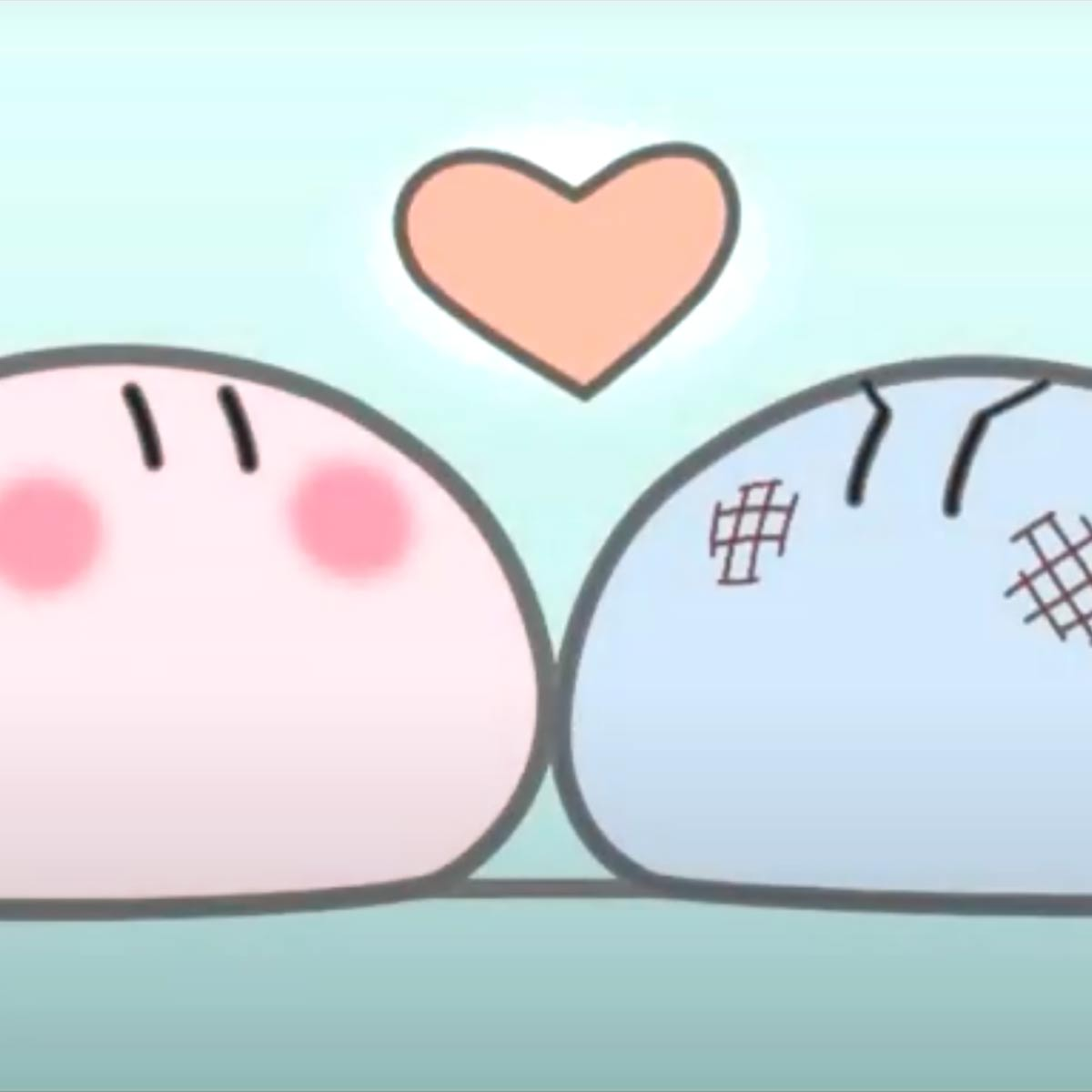# Jun Maeda

2 Music Sheets

Jun Maeda

• ###### Born:

3rd January 1975

• ###### Genres:

Jun Maeda (Maeda Jun) is a Japanese writer and co-founder of the visual novel brand Key under VisualArt's. He is considered a pioneer of nakige visual novels, and has mainly contributed as a scenario writer, lyricist, and musical composer for the games the company produces. His style was originally inspired by James Herbert Brennan, and is influenced by Haruki Murakami's novel Hard-Boiled Wonderland and the End of the World.

## Artist's Music Sheets

•c Z V V B B m V Z c Z V V B B m B c Z V V B B m V Z c Z V V B B m V g D H H J J l H D g D H H J J l J g D H H J J l H D g D H H J J l H D g D H H J J l H D g D H H J J l J g D H H J J l H D g D H H J J l H D L l H g P H J g OH D g H L li H g O H J g OH Y i O L l H g P H J g OH D g H D H S S D S D S EYP D H J J* H g W H J l Z J l YH D H J J* H g i J H Z 8l YP D H J J* H g W H J l Z J l YH th g * W T h H h % ( W O YD P H J J* H g W H J l Z J l YH D H J J* H g i J H Z 8l YP D H J J* H g W H J l Z J l YH th g * W T h H h H g D H H J J l H D g D H H J J l J g D H H J J l H D g D H H J J l H
Level: 3
Easy

#### Jun Maeda

•c Z V V B B m V Z |
c Z V V B B m m V |
c Z V V B B m V Z |
c Z V V B B V
[Tg] D H [iH] J [tJ] l [iH] D
[Tg] D H [iH] J [tJ] l [oJ]
[Tg] D H [iH] J [tJ] l [iH] D
[Tg] D H [iH] J [OH] [oD]
[Tg] D H [iH] J [tJ] l [iH] D
[Tg] D H [iH] J [tJ] l [oJ]
[Tg] D H [iH] J [tJ] l [iH] D

[Tg] D H [iH] J [OH] [oD] [IL] l H g P H J g [OH] D [Yg] H [iL] l H g O H J g [OH] Y i O [IL] l H g P H J g [OH] D [Yg] H [iD] H S S D S D S [5PJ] D [YH] J [*J] [WH] g [iTO] H [WJ] l [8Z] J [Wl] [tYOH] D [WH] J [*J] [WH] g [iTO] J [WH] Z [ql] t [oP] D [YH] J [*J] [WH] g [iTO] H [WJ] l [8Z] J [Wl] [tYOH] [Wh] [^g] q E [Tg] [[h] E [YH] [oh] % H [tY] [ [5D] D [YH] [oJ] [*J] [WH] g [iTO] H [WJ] l [8Z] J [Wl] [tYOH] D [WH] J [*J] [WH] g [iTO] J [WH] Z [ql] t [oP] D [YH] J [*J] [WH] g [iTO] H [WJ] l [8Z] J [Wl] [tYOH] [Wh] [^Sg] E [[Dh] [wg] h % H [ [tYO] [TJ] i O H g H J H Z l [tJ] Y O s H D H J H Z l [TJ] O S H g H J H Z l H J [Hql] t i t [ohJ] t P t [qgH] t [ih] t [[g] t [iD] t [WP] [TO] [Wi] [iO] [WP] [TO] [WD] s * W T W i W T W [[P] [WO] [tY] [WO] [YP] [WO] [tD] [Ws] [ W t W Y W t W [*P] [WO] [Ti] [WO] [iP] [WO] [TD] [Ws] * W T W [iO] W T W [[P] E Y o P Y [ED] o [Ph] D Z [hJ] D H J [*J] [WH] g [iTO] H [WJ] l [8Z] J [Wl] [tYOH] D [WH] J [*J] [WH] g [iTO] J [WH] Z [Hqgl] t [whJ] D [EH] J [*J] [WH] g [iTO] H [WJ] l [8Z] J [Wl] [tYOH] [Wh] [^g] q E [Tg] [[h] E [YH] [oh] % H [tY] [ [5J] D [YH] [oJ] [*J] W [TH] [Wg] [iO] [WH] [TJ] [Wl] [8Z] [WJ] [tl] W [YOH] [tD] [YH] [WJ] [*J] W [TH] [Wg] [iO] [WJ] [TH] [WZ] [4ql] t [iO] t [5wEJ] D [YH] [oJ] [*J] W [TH] [Wg] [iO] [WH] [TJ] [Wl] [8Z] [WJ] [tl] W [YOH] Y [Wh] [^g] q [ETi] q [[h] E [YH] [oh] % H [tY] [ [5J] D [YH] [oJ] [*J] W [TH] [Wg] [iO] [WH] [TJ] [Wl] [8Z] [WJ] [tl] W [YOH] [tD] [YH] [WJ] [*J] W [TH] [Wg] [iO] [WJ] [TH] [WZ] [4ql] W t [5wJ] D [YH] [oJ] [*J] W [TH] [Wg] [iO] [WH] [TJ] [Wl] [*Z] [WJ] [Tl] W [iOH] W [Th] W [^g] q E [Tg] [[h] E [YH] [oh] % [[H] W t [5J] [[D] [wH] [EJ] [*J] W [TH] [Wg] [iO] [WH] [TJ] [Wl] [8Z] [WJ] [tl] W [YOH] [tD] [YH] [WJ] [*J] W [TH] [Wg] [iO] [WJ] [TH] [WZ] [4ql] W t [5wJ] D [YH] [oJ] [*J] W [TH] [Wg] [iO] [WH] [TJ] [Wl] [8Z] [WJ] [tl] W [YOH] t [Wh] [^g] q E T [[h] E [YH] [oh] [*c] [WZ] [TV] Y [iV] B B m V Z c Z V V B B m V c Z V V B B m V Z c Z V V B V c Z V V B B m V Z c Z V V B B m V c Z V V B B m V Z c Z V V B V

Level: 6
Intermediate